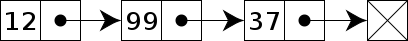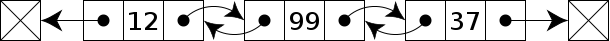# Implement Linked List in Ruby1. Introduction
2. What is a linked list
3. Why/When to use a linked list
5. Conclusion

## What is a linked list

A linked list is a data structure used in computer science and programming to store and manage a collection of elements called nodes. Unlike arrays, which store elements in contiguous memory locations, a linked list consists of nodes that are individually allocated in memory and connected together through references or pointers.

Each node in a linked list contains two components: the data or value of the node, and a reference or pointer to the next node in the sequence. The last node in the list typically has a null reference, indicating the end of the list.

Here's an example of a simple linked list with three nodes:

``````Node 1       Node 2       Node 3
+-------+    +-------+    +-------+
|  Data |    |  Data |    |  Data |
|-------|    |-------|    |-------|
|  Next ------> Next ------> Null  |
+-------+    +-------+    +-------+
``````

To traverse a linked list, you start from the head or first node and follow the next references until you reach the end of the list. Inserting or deleting nodes in a linked list involves adjusting the references or pointers to maintain the correct order and connections.

Linked lists have certain advantages and disadvantages compared to other data structures. They allow for efficient insertion and deletion operations at the beginning or middle of the list but have slower access times for random elements compared to arrays. Additionally, linked lists can dynamically grow or shrink in size without requiring contiguous memory blocks, unlike arrays.

## Why/When to use a linked list

So when should we use a linked list data structure, well a linked list should be used shen changes to a collection need to be made very often like addition and deletion operations. In this case Linkedlist is faster than using the Array method.

In Ruby, you can implement a linked list using classes and objects. Here's an example of a basic implementation of a singly linked list:First we create a class for the node and initialize the data and the pointer(next).

``````class Node
attr_accessor :data, :next

def initialize(data)
@data = data
@next = nil
end
end
``````

Then we create a class for the linked list and initialize the head of the list as nil. This class will be used to hold the methods that will add/remove and insert a node to the list.

``````class LinkedList
def initialize
end
``````

Here we add our first method `append` to our `Linkedlist` class, we create a `new_node` that will be the value of the (data) we want to add and then we say if the head of our list is nil then the head will be our `new_node` else the next node will be our new_node `current.next = new_node`, this method will add a node to the end of our list.

`````` ----previous code----
def append(data)
new_node = Node.new(data)
else
current = current.next until current.next.nil?
current.next = new_node
end
end
``````

The next method we will add is the `prepend` method, this will add a node to the top of our list making the node the head. We again declear a `new_node` and say if the head is nil our `new_node` will be the head but if the head isn't nil the `new_node` we are about to add will then become the head `new_node.next = @head`

``````----previous code----
def prepend(data)
new_node = Node.new(data)
else
end
end
``````

Next we add the `insert_after` method this takes a position and data(new_node) as arguments, we say if the position argument is equal to the number 0 then the `new_node` becomes the head but if our position is greater than 0 then the node will be inserted after that position(we dont count the first node as 0, in the case we count is as 1) `current.next = new_node`

``````----previous code----
def insert_after(position, data)
new_node = Node.new(data)

if position == 0
else
(position - 1).times do
break if current.nil?

current = current.next
end

return if current.nil?

new_node.next = current.next
current.next = new_node
end
end
``````

For the delete method we say if the head is the same as the data we want to remove then the head is actually the next element in line `@head = @head.next` but if the element we want to delete is the next in line the we just skip it and we get the element after it
`current.next = current.next.next unless current.next.nil?`

``````----previous code----
def delete(data)

else
while current.next && current.next.data != data
current = current.next
end

current.next = current.next.next unless current.next.nil?
end
end
``````

Finally here we add the method that will display all the information in the terminal from the head of the linkedlist to the tail.

``````----previous code----
def display
while current
puts current.data
current = current.next
end
end
end
``````

Here's an example of how you can use the LinkedList class:

``````list = LinkedList.new

list.append(1)
list.append(2)
list.append(3)

list.prepend(0)

list.insert_after(2, 1.5)

list.display
``````

This will output:

``````0
1
1.5
2
3
``````

In this example, we first create a `Node` class to represent a node in the linked list. Each node has a `data` attribute to store the value and a `next` attribute to reference the next node.

Then, we create a `LinkedList` class that manages the linked list. It has methods like `append` to add a node at the end, `prepend` to add a node at the beginning, `insert_after` to insert a node after a given position, `delete` to remove a node with a specific value, and `display` to print the elements of the linked list.

Now we will show you a doubly linked list, there is not much difference to this implementation. We just add a prev pointer that points to the previous node.First we create a class for the node and initialize the data, the pointer(next) and the pointer(prev).Then we create a class for the linked list and initialize the head and the tail of the list as nil. This class will be used to hold all the methods as the previous linked list plus and additional one that prints the list in reverse.

``````class Node
attr_accessor :data, :next, :prev

def initialize(data)
@data = data
@next = nil
@prev = nil
end
end

def initialize
@tail = nil
end
``````

As with the append and prepend method in the first linked list the difference is with the append the node added will be the `@tail.next = new_node` and with the prepend the node added is the `@head.prev = new_node`.

``````----previous code----
def append(data)
new_node = Node.new(data)
@tail = new_node
else
new_node.prev = @tail
@tail.next = new_node
@tail = new_node
end
end

def prepend(data)
new_node = Node.new(data)
@tail = new_node
else
end
end
``````

We have some slight differences here from the first `insert_after` method we made provisions for the @tail to become the new node if needed `@tail = new_node if @tail.nil?` `@tail = new_node if new_node.next.nil?`

``````----previous code----
def insert_after(position, data)
new_node = Node.new(data)

if position == 0
@tail = new_node if @tail.nil?
else
(position - 1).times do
break if current.nil?

current = current.next
end

return if current.nil?

new_node.next = current.next
new_node.prev = current
current.next = new_node
new_node.next.prev = new_node if new_node.next
@tail = new_node if new_node.next.nil?
end
end
``````

The delete method is pretty much of the same, difference is we made adjustments for the tail and said `@tail= current.prev if current == @tail` if the tail element is the one removed then the previous element becomes the tail.

``````----previous code----
def delete(data)

while current && current.data != data
current = current.next
end

return if current.nil?

else
current.prev.next = current.next
current.next.prev = current.prev if current.next
end

@tail = current.prev if current == @tail
end
``````

Here the first display method is still the same, but we can now add a method to display the numbers in reverse starting from the tail we just change `current` variable to equal the tail and point to the `current.prev`

``````----previous code----
def display_forward
while current
puts current.data
current = current.next
end
end

def display_backward
current = @tail
while current
puts current.data
current = current.prev
end
end
end
``````

``````list = DoublyLinkedList.new

list.append(1)
list.append(2)
list.append(3)

list.prepend(0)

list.insert_after(2, 1.5)

list.display_forward
puts "----"
list.display_backward
``````

This is the result

``````0
1
1.5
2
3
----
3
2
1.5
1
0
``````

You can create an instance of the `LinkedList` class and use its methods to manipulate the linked list as shown in the example. The completed code should look like this when you put it all together.

``````class Node
attr_accessor :data, :next

def initialize(data)
@data = data
@next = nil
end
end

def initialize
end

def append(data)
new_node = Node.new(data)
else
current = current.next until current.next.nil?
current.next = new_node
end
end

def prepend(data)
new_node = Node.new(data)
else
end
end

def insert_after(position, data)
new_node = Node.new(data)

if position == 0
else
(position - 1).times do
break if current.nil?

current = current.next
end

return if current.nil?

new_node.next = current.next
current.next = new_node
end
end

def delete(data)

else
while current.next && current.next.data != data
current = current.next
end

current.next = current.next.next unless current.next.nil?
end
end

def display
while current
puts current.data
current = current.next
end
end
end
``````

``````class Node
attr_accessor :data, :next, :prev

def initialize(data)
@data = data
@next = nil
@prev = nil
end
end

def initialize
@tail = nil
end

def append(data)
new_node = Node.new(data)
@tail = new_node
else
new_node.prev = @tail
@tail.next = new_node
@tail = new_node
end
end

def prepend(data)
new_node = Node.new(data)
@tail = new_node
else
end
end

def insert_after(position, data)
new_node = Node.new(data)

if position == 0
@tail = new_node if @tail.nil?
else
(position - 1).times do
break if current.nil?

current = current.next
end

return if current.nil?

new_node.next = current.next
new_node.prev = current
current.next = new_node
new_node.next.prev = new_node if new_node.next
@tail = new_node if new_node.next.nil?
end
end

def delete(data)

while current && current.data != data
current = current.next
end

return if current.nil?

else
current.prev.next = current.next
current.next.prev = current.prev if current.next
end

@tail = current.prev if current == @tail
end

def display_forward
while current
puts current.data
current = current.next
end
end

def display_backward
current = @tail
while current
puts current.data
current = current.prev
end
end
end
``````

## Conclusion

So we have learned when you can use a linked list, how to implement it using Ruby and also added some methods you can use to add a node to the begining, middle and end of the list and also delete a node.